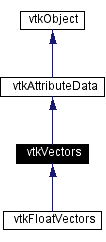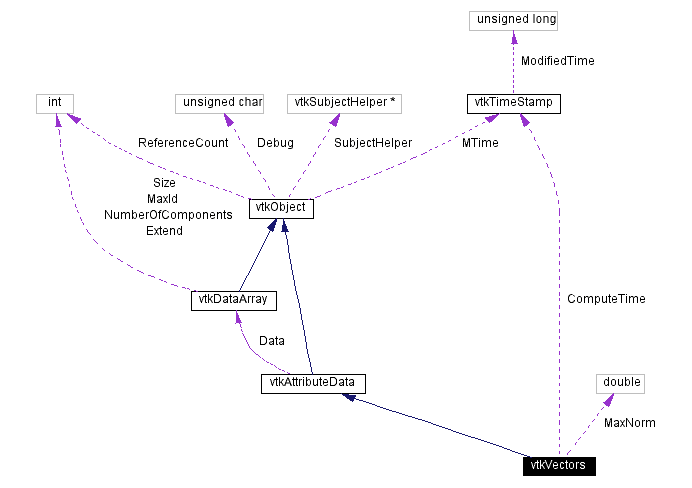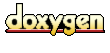Main Page   Class Hierarchy   Alphabetical List   Compound List   File List   Compound Members   File Members   Related Pages

# vtkVectors Class Reference

represent and manipulate 3D vectors. More...

`#include <vtkVectors.h>`

Inheritance diagram for vtkVectors:[legend]
Collaboration diagram for vtkVectors:[legend]
List of all members.

## Public Methods

virtual const char * GetClassName ()
virtual int IsA (const char *type)
void PrintSelf (ostream &os, vtkIndent indent)
vtkAttributeDataMakeObject ()
int GetNumberOfVectors ()
float * GetVector (int id)
void GetVector (int id, float v)
void GetVector (int id, double v)
void SetVector (int id, const float v)
void SetVector (int id, const double v)
void SetVector (int id, double vx, double vy, double vz)
void InsertVector (int id, const float v)
void InsertVector (int id, const double v)
void InsertVector (int id, double vx, double vy, double vz)
int InsertNextVector (const float v)
int InsertNextVector (const double v)
int InsertNextVector (double vx, double vy, double vz)
void SetNumberOfVectors (int number)
void ComputeMaxNorm ()
double GetMaxNorm ()
void GetVectors (vtkIdList *ptId, vtkVectors *fv)
void GetVectors (vtkIdList &ptId, vtkVectors &fv)

## Static Public Methods

vtkVectors * New (int dataType)
vtkVectors * New ()
int IsTypeOf (const char *type)
vtkVectors * SafeDownCast (vtkObject *o)

## Protected Methods

vtkVectors ()
~vtkVectors ()
vtkVectors (const vtkVectors &)
void operator= (const vtkVectors &)

## Protected Attributes

double MaxNorm
vtkTimeStamp ComputeTime

## Detailed Description

represent and manipulate 3D vectors.

Date:
2000/12/10 20:08:20
Revision:
1.51

vtkVectors represents 3D vectors. The data model for vtkVectors is an array of vx-vy-vz triplets accessible by (point or cell) id.

Examples:
vtkVectors (examples)

Definition at line 61 of file vtkVectors.h.

## Constructor & Destructor Documentation

 vtkVectors::vtkVectors ( ) ` [protected]`

 vtkVectors::~vtkVectors ( ) ` [inline, protected]`
 Definition at line 130 of file vtkVectors.h.

 vtkVectors::vtkVectors ( const vtkVectors & ) ` [inline, protected]`
 Definition at line 131 of file vtkVectors.h.

## Member Function Documentation

 vtkVectors* vtkVectors::New ( int dataType ) ` [static]`

 vtkVectors* vtkVectors::New ( ) ` [static]`
 Create an object with Debug turned off, modified time initialized to zero, and reference counting on. Reimplemented from vtkObject. Reimplemented in vtkFloatVectors. Referenced by MakeObject().

 virtual const char* vtkVectors::GetClassName ( ) ` [virtual]`
 Return the class name as a string. This method is defined in all subclasses of vtkObject with the vtkTypeMacro found in vtkSetGet.h. Reimplemented from vtkAttributeData.

 int vtkVectors::IsTypeOf ( const char * type ) ` [static]`
 Return 1 if this class type is the same type of (or a subclass of) the named class. Returns 0 otherwise. This method works in combination with vtkTypeMacro found in vtkSetGet.h. Reimplemented from vtkAttributeData.

 virtual int vtkVectors::IsA ( const char * type ) ` [virtual]`
 Return 1 if this class is the same type of (or a subclass of) the named class. Returns 0 otherwise. This method works in combination with vtkTypeMacro found in vtkSetGet.h. Reimplemented from vtkAttributeData.

 vtkVectors* vtkVectors::SafeDownCast ( vtkObject * o ) ` [static]`
 Will cast the supplied object to vtkObject* is this is a safe operation (i.e., a safe downcast); otherwise NULL is returned. This method is defined in all subclasses of vtkObject with the vtkTypeMacro found in vtkSetGet.h. Reimplemented from vtkAttributeData.

 void vtkVectors::PrintSelf ( ostream & os, vtkIndent indent ) ` [virtual]`
 Methods invoked by print to print information about the object including superclasses. Typically not called by the user (use Print() instead) but used in the hierarchical print process to combine the output of several classes. Reimplemented from vtkAttributeData.

 vtkAttributeData* vtkVectors::MakeObject ( ) ` [inline, virtual]`
 Create the same type object as this (virtual constructor). Reimplemented from vtkAttributeData. Definition at line 71 of file vtkVectors.h.

 int vtkVectors::GetNumberOfVectors ( ) ` [inline]`
 Return number of vectors in array. Definition at line 75 of file vtkVectors.h.

 float* vtkVectors::GetVector ( int id ) ` [inline]`
 Return a pointer to a float vector v for a specific id. Definition at line 78 of file vtkVectors.h.

 void vtkVectors::GetVector ( int id, float v ) ` [inline]`
 Copy vector components into user provided array v for specified id. Definition at line 82 of file vtkVectors.h.

 void vtkVectors::GetVector ( int id, double v ) ` [inline]`
 Definition at line 83 of file vtkVectors.h.

 void vtkVectors::SetVector ( int id, const float v ) ` [inline]`
 Insert vector into object. No range checking performed (fast!). Make sure you use SetNumberOfVectors() to allocate memory prior to using SetVector(). Definition at line 88 of file vtkVectors.h.

 void vtkVectors::SetVector ( int id, const double v ) ` [inline]`
 Definition at line 89 of file vtkVectors.h.

 void vtkVectors::SetVector ( int id, double vx, double vy, double vz ) ` [inline]`
 Definition at line 146 of file vtkVectors.h.

 void vtkVectors::InsertVector ( int id, const float v ) ` [inline]`
 Insert vector into object. Range checking performed and memory allocated as necessary. Definition at line 94 of file vtkVectors.h.

 void vtkVectors::InsertVector ( int id, const double v ) ` [inline]`
 Definition at line 96 of file vtkVectors.h.

 void vtkVectors::InsertVector ( int id, double vx, double vy, double vz ) ` [inline]`
 Definition at line 155 of file vtkVectors.h.

 int vtkVectors::InsertNextVector ( const float v ) ` [inline]`
 Insert vector into next available slot. Returns id of slot. Definition at line 101 of file vtkVectors.h.

 int vtkVectors::InsertNextVector ( const double v ) ` [inline]`
 Definition at line 103 of file vtkVectors.h.

 int vtkVectors::InsertNextVector ( double vx, double vy, double vz ) ` [inline]`
 Definition at line 165 of file vtkVectors.h.

 void vtkVectors::SetNumberOfVectors ( int number ) ` [inline]`
 Specify the number of vectors for this object to hold. Does an allocation as well as setting the MaxId ivar. Used in conjunction with SetVector() method for fast insertion. Definition at line 140 of file vtkVectors.h.

 void vtkVectors::ComputeMaxNorm ( )
 Compute the largest norm for these vectors.

 double vtkVectors::GetMaxNorm ( )
 Return the maximum norm for these vectors.

 void vtkVectors::GetVectors ( vtkIdList * ptId, vtkVectors * fv )
 Given a list of pt ids, return an array of vectors.

 void vtkVectors::GetVectors ( vtkIdList & ptId, vtkVectors & fv ) ` [inline]`
 For legacy compatibility. Do not use. Definition at line 123 of file vtkVectors.h.

 void vtkVectors::operator= ( const vtkVectors & ) ` [inline, protected]`
 Definition at line 132 of file vtkVectors.h.

## Member Data Documentation

 double vtkVectors::MaxNorm` [protected]`
 Definition at line 134 of file vtkVectors.h.

 vtkTimeStamp vtkVectors::ComputeTime` [protected]`
 Definition at line 135 of file vtkVectors.h.

The documentation for this class was generated from the following file:

Generated on Wed Nov 21 13:01:28 2001 for VTK by1.2.11.1 written by Dimitri van Heesch, © 1997-2001